Homework Help Question & Answers

# In the figure, a region with constant (externally produced) magnetic field is shown. A wire loop...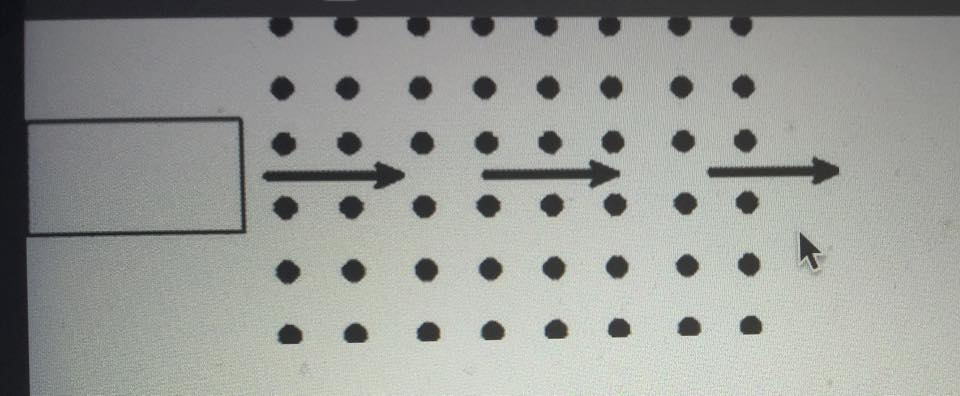In the figure, a region with constant (externally produced) magnetic field is shown. A wire loop moves into the magnetic field.//img.homeworklib.com/questions/b9da6130-ca78-11eb-8c71-8b0cea09251c.gif?x-oss-process=image/resize,w_560

Which of the following is correct? While the loop is moving into the region with the constant external magnetic field, the magnetic field inside the loop due to the induced current in the loop points ...
No magnetic field is induced by the movement of the loop.
... down
... left
... into the page
... right
... up
... out of the page (the answer)

 Tries 0/20

Which of the following is correct? While the loop is moving into the region with the constant external magnetic field, the magnetic force on the loop points ...
... right
... up
There is no magnetic force acting on the loop.
... out of the page
... down
... left
... into the page

I need help with the last 2 problems! Please explain as well!

#### Homework Answers

Answer #1

From Faraday's law of induction we know, The induced electromotive force in any closed circuit is equal to the negative of the time rate of change of the magnetic flux enclosed by the circuit. Here we have a constant magnetic field B acting inside the page. The loop has a constant area A. The magnetic flux through the loop, when it passes the region of constant magnetic field is,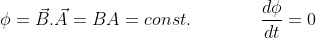This means no emf is generated along the loop. Hence no induced current and no induced magnetic field is generated.

We know that magnetic force acts on a current carrying loop, which is given by,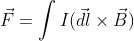Here the loop is not carrying any current (I = 0), not real neither induced. Hence magnetic force acting on the loop is zero.

Know the answer?
Your Answer:

#### Post as a guest

Your Name:

What's your source?

#### Earn Coin

Coins can be redeemed for fabulous gifts.

Not the answer you're looking for? Ask your own homework help question. Our experts will answer your question WITHIN MINUTES for Free.
Similar Homework Help Questions
• ### MULTIPLE CHOICE - Please help!!! Urgent - Wire Loop Right Hand Rule - Will Rate!!! One part...In the figure, a region withconstant (externally produced) magnetic field is shown. A wire loopmoves into the magnetic field.Which of the following is correct? While the loop ismoving into the region with the constant external magnetic field,the magnetic field inside the loopdue to the induced current inthe loop points ...Incorrect:... rightCorrect:... into the pageIncorrect:... downIncorrect:... upIncorrect:No magnetic field is induced by the movement ofthe loop.Incorrect:... leftIncorrect:... out of the pageWhich of the followingis correct? While the loop is moving into...

• ### For example, consider the situation shown on the right in which a rectangular loop is 8...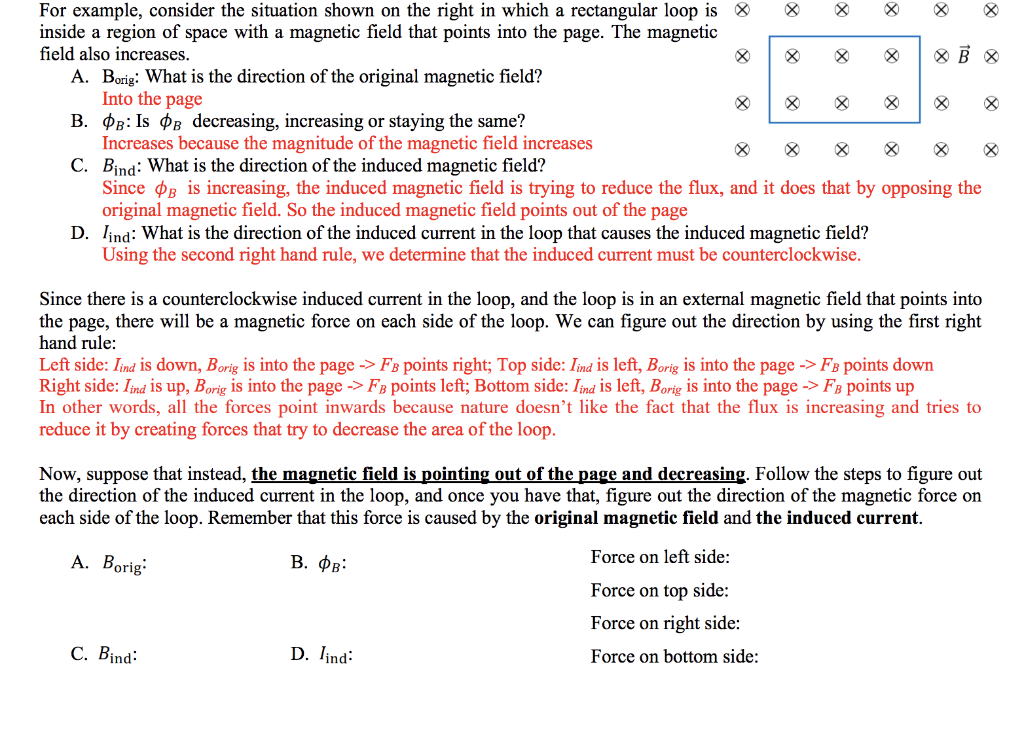For example, consider the situation shown on the right in which a rectangular loop is 8 inside a region of space with a magnetic field that points into the page. The magnetic field also increases. A. Borig: What is the direction of the original magnetic field? Into the page B. PB: Is ºb decreasing, increasing or staying the same? Increases because the magnitude of the magnetic field increases C. Bind: What is the direction of the induced magnetic field? Since...

• ### In a region with a constant magnetic field as shown, there are two conducting frictionless horizontal...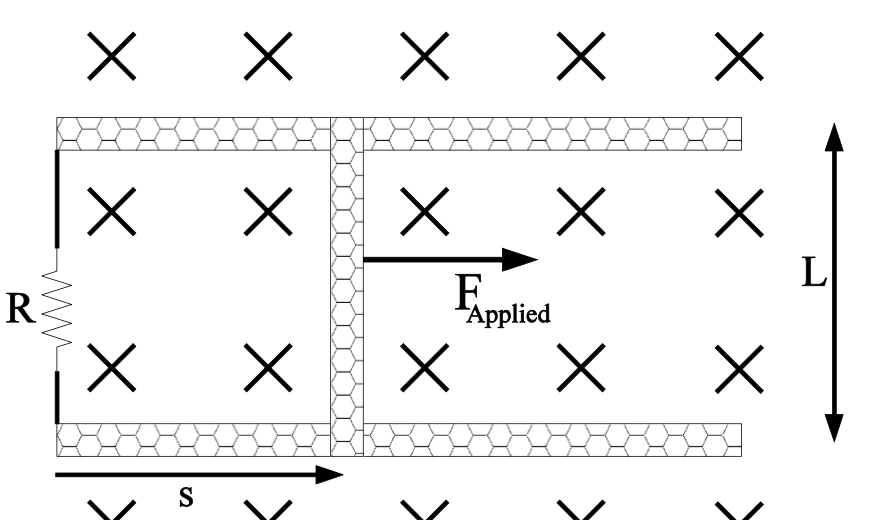In a region with a constant magnetic field as shown, there are two conducting frictionless horizontal rails, a resistor and some conducting wire along the left side and a conducting bar on the right side that is free to slide left or right. The bar is initially at rest but at time zero a force is applied to the bar pulling it to the right so that the distance s increases with time. a. Magnetic flux through the loop increases...

• ### B A loop is moving from a region with no magnetic field to a region with...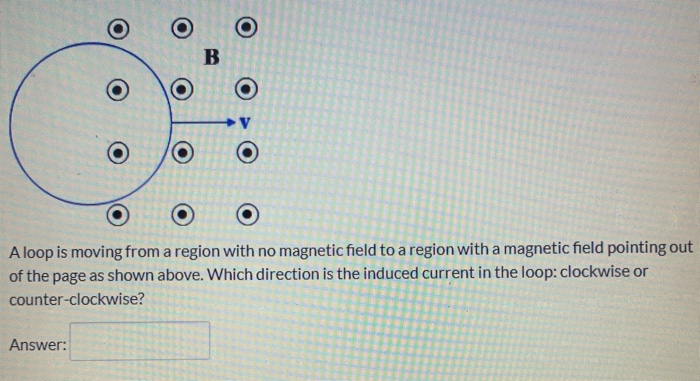B A loop is moving from a region with no magnetic field to a region with a magnetic field pointing out of the page as shown above. Which direction is the induced current in the loop: clockwise or counter-clockwise? Answer:

• ### 1. A rectangular coil of wire with sides W and H has N turns. To its right is a region of uniform...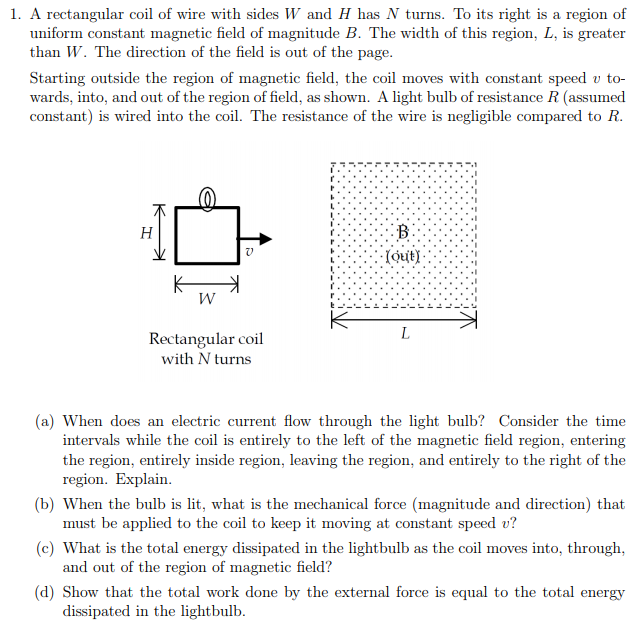1. A rectangular coil of wire with sides W and H has N turns. To its right is a region of uniform constant magnetic field of magnitude B. The width of this region, L, is greater than W. The direction of the field is out of the page Starting outside the region of magnetic field, the coil moves with constant speed v to- wards, into, and out of the region of field, as shown. A light bulb of resistance R...

• ### A loop of wire is at the edge of a region of space containing auniform magnetic...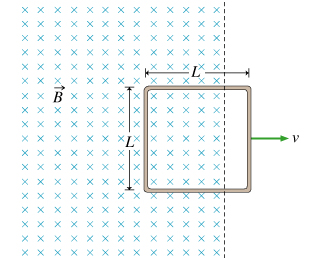A loop of wire is at the edge of a region of space containing auniform magnetic field . The plane of the loop isperpendicular to the magnetic field. Now the loop is pulled out ofthis region in such a way that the area of the coil inside the magnetic field region isdecreasing at the constant rate . That is, , with . Part AThe induced emf in the loop is measuredto be . What is the magnitude of the magnetic...

• ### A wire loop travels to the right at a constant velocity. Which plot best represents the...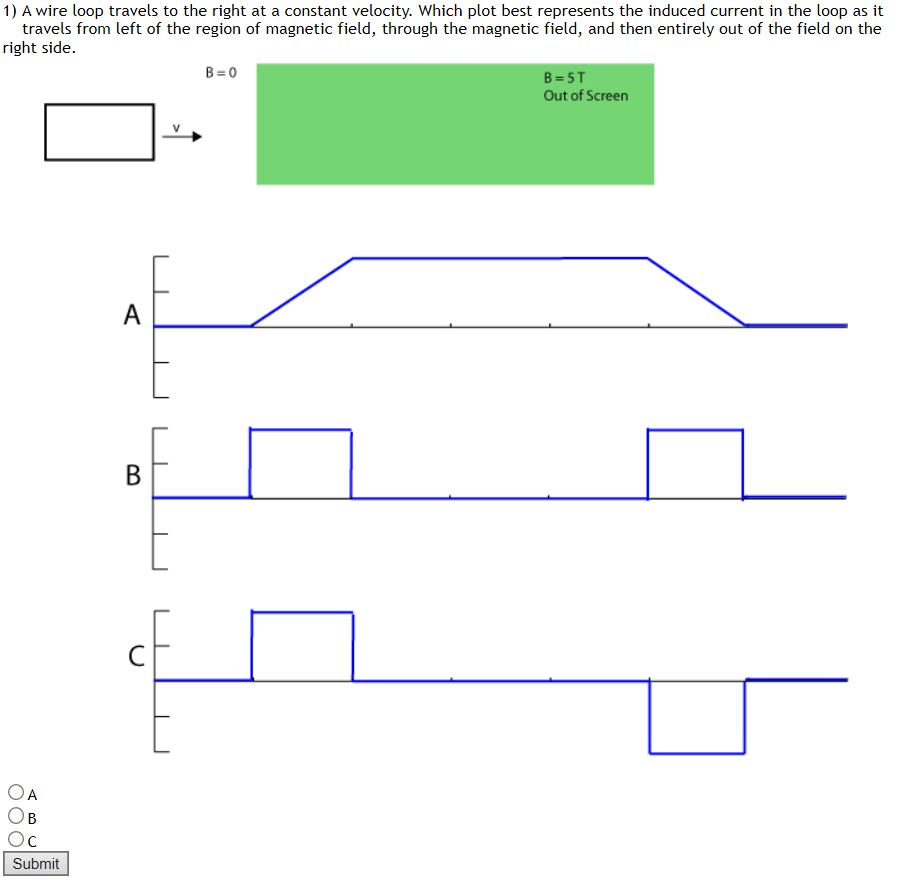A wire loop travels to the right at a constant velocity. Which plot best represents the induced current in the loop as it travels from left of the region of magnetic field, through the magnetic field, and then entirely out of the field on the right side? A B C 1) A wire loop travels to the right at a constant velocity. Which plot best represents the induced current in the loop as it travels from left of the region...

• ### Magnetic field region There is a uniform magnetic field of magnitude B= 1.6T and directed out...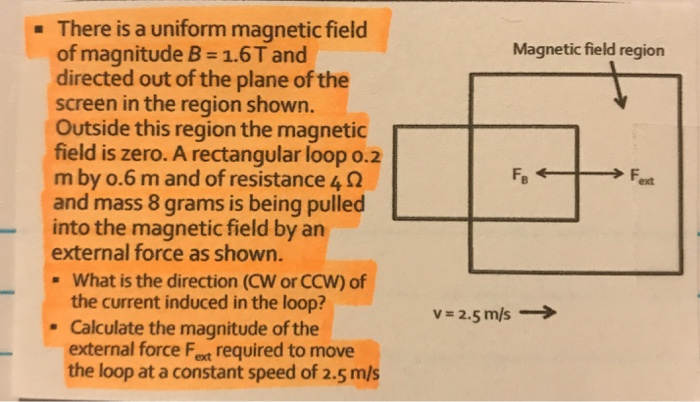Magnetic field region There is a uniform magnetic field of magnitude B= 1.6T and directed out of the plane of the screen in the region shown. Outside this region the magnetic field is zero. A rectangular loop o.2 mby 0.6 m and of resistance 42 and mass 8 grams is being pulled into the magnetic field by an external force as shown. - What is the direction (CW or CCW) of the current induced in the loop? Calculate the magnitude...

• ### a. Consider a circular conducting loop that is moved at a constant speed from right to...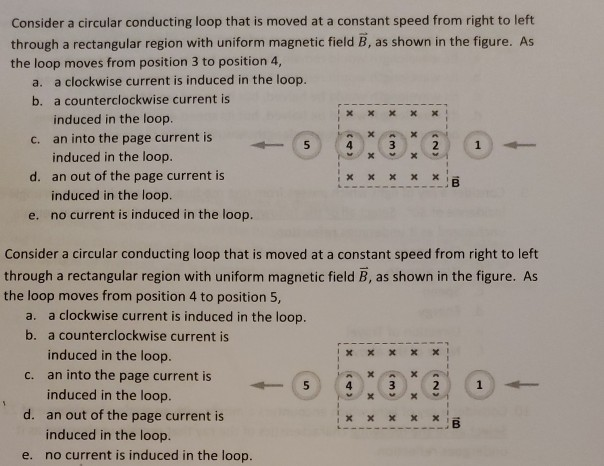a. Consider a circular conducting loop that is moved at a constant speed from right to left through a rectangular region with uniform magnetic field B, as shown in the figure. As the loop moves from position 3 to position 4, a clockwise current is induced in the loop. b. a counterclockwise current is x x induced in the loop. an into the page current is 5 1 induced in the loop. d. an out of the page current is...

Free Homework App

Scan Your Homework
to Get Instant Free Answers
Need Online Homework Help?

Get Answers For Free
Most questions answered within 3 hours.